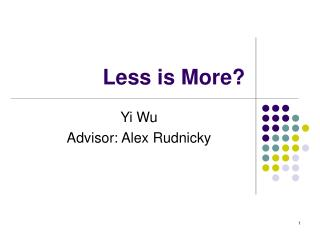DownloadDownload PresentationLess is More?

# Less is More?

Télécharger la présentation## Less is More?

- - - - - - - - - - - - - - - - - - - - - - - - - - - E N D - - - - - - - - - - - - - - - - - - - - - - - - - - -
##### Presentation Transcript

1. Less is More? Yi Wu Advisor: Alex Rudnicky

2. People: There is no data like more data!

3. Goal: Use less to Perform more • Identifying an informative subset from a large corpus for Acoustic Model (AM) training. • Expectation of the Selected Set • Good in Performance • Fast in Selection

4. Motivation • The improvement of system will become increasingly smaller when we keep adding data. • Training acoustic model is time consuming. • We need some guidance on what is the most needed data.

5. Approach Overview • Applied to well-transcribed data • Selection based on transcription • Choose subset that have “uniform” distribution on speech unit (word, phoneme, character)

6. k Gaussian distribution with known priorωi and unknown density function fi(μi ,σi) How to sample data wisely?--A simple example

7. How to sample wisely?--A simplified example • We are given access to at most N examples. • We have right to choose how much we want from each class. • We train the model use MLE estimator. • When a new sample generated, we use our model to determine its class. Question: How to sample to achieve minimum error?

8. The optimal Bayes Classifier If we have the exact form of fi(x), above classification is optimal.

9. To approximate the optimal • We use our MLE • The true error would be bounded by optimal Bayes error plus error bound for our worst estimated

10. Sample Uniformly • We want to sample each class equally. • The data selected will have good coverage on each class. • This will give robust estimation on each class.

11. The Real ASR system

12. Data Selection for ASR System • The prior has been estimated independently by language model. • To make acoustic model accurate, we want to sample the W uniformly. • We can take the unit to be phoneme, character, word. We want their distribution to be uniform.

13. Entropy: Measure for “uniformness” • Use the entropy of the word (phoneme) as ways of evaluation • Suppose the word (phoneme) has a sample distribution p1, p2…. pn • Choose subset have maximum -p1*log(p1)-p2*log(p2)-... pn *log(pn)) • Entropy actually is the KL distance from uniform distribution

14. Computational Issue • It is computational intractable to find the transcription set that maximizes the entropy • Forward Greedy Search

15. Combination • There are multiple entropies we want to maximize. • Combination Method • Weighted Sum • Add sequentially

16. Experiment Setup • System: Sphinx III • Feature: 39 dimension MFCC • Training Corpus: Chinese BN 97(30hr)+ GaleY1(810hr data) • Test Set: RT04(60 min)

17. More Result

18. Experiment 1,2

19. Experiment 3 (with VTLN) Table 3

20. Summary • Choose data uniformly according to speech unit • Maximize entropy using greedy algorithm • Add data sequentially Future Work • Combine Multiple Sources • Select Un-transcribed Data